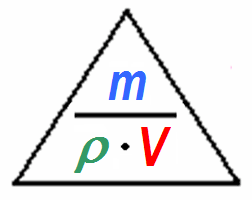Deutsche Versionprefixes | length | area | volume | weight | pressure | temperature | time | energy | power | velocity | acceleration | force

Conversions of density units
Density units conversion of ρ = m / V
This program converts English and American measures to metric units
 kilograms per cubic meter (kg/m³)  = gram per liter (g/L or g/dm³) kilograms per liter (kg/L or kg/dm³)  = tons per cubic meter (t/m³)   = grams per cubic centimeter (g/cm³)
 pounds per cubic yard pounds per cubic foot long ton per cubic yard pounds per cubic inch

Density = mass / volume      ρ = m / V

Please enter two values, the third value will be calculated.

 Density ρ kg/m³Mass m kg Volume V m³

You can also enter the density in g/cm³, the mass in g, and the volume in cm³.

Conversions of many more density units

Density conversion factors from non-SI units

 Unit Symbol Equivalent SI value pounds per cubic foot lb / ft3 16.018 46 kg / m3 slugs per cubic foot slug / ft3 515.378 8 kg / m3 pounds per cubic inch lb / in3 27 679.90 kg / m3

 Many people still use g/cm3 (gram per cubic centimeter) or kg/L (kilograms per liter) to measure density. SI standard is kg/m3. 1 kg/dm3 = 1000 g/1000 cm3 = 1 kg/L. Water is the reference with its highest density at 3.98°C (ρ = 1 g/cm3) The correct SI unit of denstity of water is "ρ = 1000 kg/m3". 1 m3 = 1,000,000 cm3. Examples: Solid - water - noble gas Copper has a density of 8950 kg/m3 = 8.95 kg/dm3 = 8.95 g/cm3. Water has a density of 1000 kg/m3 = 1000 g/L = 1 kg/dm3 = 1 kg/L = 1 g/cm3 = 1 g/mL. Helium has a density of 0.1785 kg/m3 = 0.1785 g/L = 0.0001785 kg/dm3 = 0.0001785 kg/L = 0.0001785 g/cm3 = 0.0001785 g/mL. From 1901 to 1964, a litre was defined as exactly the volume of 1 kg of water at maximum density, and the maximum density of pure water was 1.000 kg/L. The maximum density of pure water at a pressure of one standard atmosphere is 999.972 kg/m3 at a temperature of 3.98 °C (277.13 K), that is 0.999 972 kg/L.

Calculation of water density (density of water H2O)

 Water temperature °C Water salinity (TDS) mg/L or ppm Water density ρ kg/m3
 Sea water has 30000 to 40000 ppm (parts per million) = mg/L salinity. TDS = Total Dissolved Solids, e.g. 3.5 % = 35 ppt = 35000 ppm. Some dinosours are still asking: What is the density of water in English units? Ok, if you really need it: The density of water is roughly 62.4 lb per cubic foot. The density of water varies somewhat with temperature and pressure, and is higher for salt water than fresh water, 1 pound per cubic foot = 16.0184634 kg / m3 and 1 kg/m3 = 0.0624279605 pounds per cubic foot.

Density of water and the dependence on temperature

 Density of Water and the Dependence on the Temperature  |  3.98°C ρ = 999.975 Temp.(°C)  ρ (kg/m3) Temp.(°C)  ρ (kg/m3) 0               999.84 27               996.51 1               999.90 28               996.23 2               999.94 29               995.94 3               999.96 30               995.64 4           ● 999.97 31               995.34 5               999.96 32               995.02 6               999.94 33               994.70 7               999.90 34               994.37 8               999.85 35               994.03 9               999.78 36               993.68 10               999.70 37               993.32 11               999.60 38               992.96 12               999.50 39               992.59 13               999.38 40               992.21 14               999.24 45               990.21 15               999.10 50               988.03 16               998.94 55               985.69 17               998.77 60               983.19 18               998.59 65               980.55 19               998.40 70               977.76 20               998.20 75               974.84 21               997.99 80               971.79 22               997.77 85               968.61 23               997.54 90               965.30 24               997.29 95               961.88 25               997.04 100              958.35 26               996.78 Ice 0°C        916,80

Tabular value ÷ 1000 = density in kg/dm3 or in kg/L or in g/cm3 or in g/mL

Calculations of air density (density of air)